Lists and Tables of Data

Importing an columns of data from an ASCII file

Suppose you have matrix of data in an ASCII file, and the file is in a
directory included in \$Path.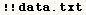In version 4.0 and later data can be brought in with the Import command using
the line in the next cell.  When the second argument is "Table" entries are
brought in as numbers when possible and as strings when they can't be
numbers.

MyData=Import["data.txt","Table"];

TableForm[MyData]

This can be done in any version of Mathematica using ReadList as below. You
might also prefer this method because it may be faster than Import. In this
example the second argument {Word, Number, Number} indicates each line is an
ASC string, followed two numbers.

TableForm[MyData]

Working with a List or Table of data

For some efficient ways of making lists see examples of Range, Array, and
Table.  Some good ways to work with a matrix of numbers are shown in the
Cells below.  First we make a matrix of random integers called (data).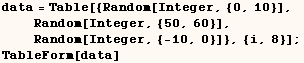2 51 -1 7 50 -5 10 57 -5 7 60 -10 6 54 -4 2 50 0 10 58 0 10 54 -10

Functions that have the Listable attribute are performed on each element  of nested lists.  Hence the following line adds 100 to each element of data.  Besides being very concise this is the most efficient way to do something  like this in Mathematica.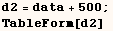502 551 499 507 550 495 510 557 495 507 560 490 506 554 496 502 550 500 510 558 500 510 554 490

In the next line MapAt is used to add 500 to the fourth row of data.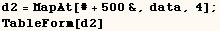2 51 -1 7 50 -5 10 57 -5 507 560 490 6 54 -4 2 50 0 10 58 0 10 54 -10

We can perform an operation f[] on the third column of data.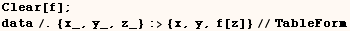2 51 f[-1] 7 50 f[-5] 10 57 f[-5] 7 60 f[-10] 6 54 f[-4] 2 50 f 10 58 f 10 54 f[-10]

Sometimes the simple method above doesn't do what you want. For example in
the next cell the pattern matcher assigns (x,y,z) to the 1st, 2nd, 3rd rows,
but we wanted (x,y,z) to be the 1st, 2nd and 3rd elements of each row.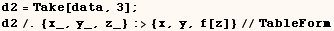2 51 -1 7 50 -5 f[{10, 57, -5}]

The problem in the last example can be avoided if we ensue either x, y or z
is numeric as in the next cell.  We could just as well ensure x, y, or z is
not a vector.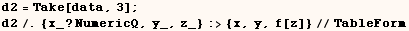2 51 f[-1] 7 50 f[-5] 10 57 f[-5]

We can swap the second and third columns. To keep this example simple I don't
ensure that neither x, y or z are numeric, but in some cases that would be
necessary.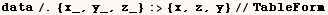2 -1 51 7 -5 50 10 -5 57 7 -10 60 6 -4 54 2 0 50 10 0 58 10 -10 54

We can select the elements of data that have a second element less than 56.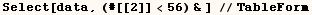2 51 -1 7 50 -5 6 54 -4 2 50 0 10 54 -10

The method below is less cryptic, but it requires the use of a global
variable to name the testing function. It also turns out that the less
cryptic method takes about twice as much time to evaluate.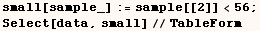2 51 -1 7 50 -5 6 54 -4 2 50 0 10 54 -10

One method that's very attractive is to use Cases as below.
Also see the  separate discussion of Cases.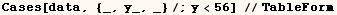2 51 -1 7 50 -5 6 54 -4 2 50 0 10 54 -10

To see how to sort a matrix according to the values in a certain column of  the matrix see the discussion of Sort.  For more on this topic see